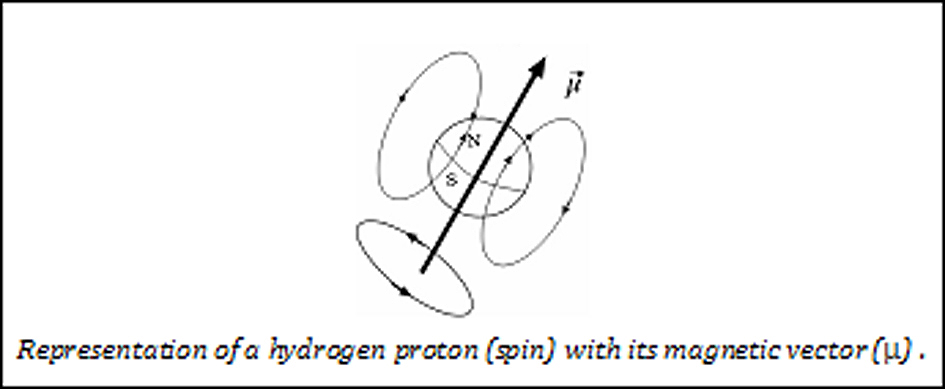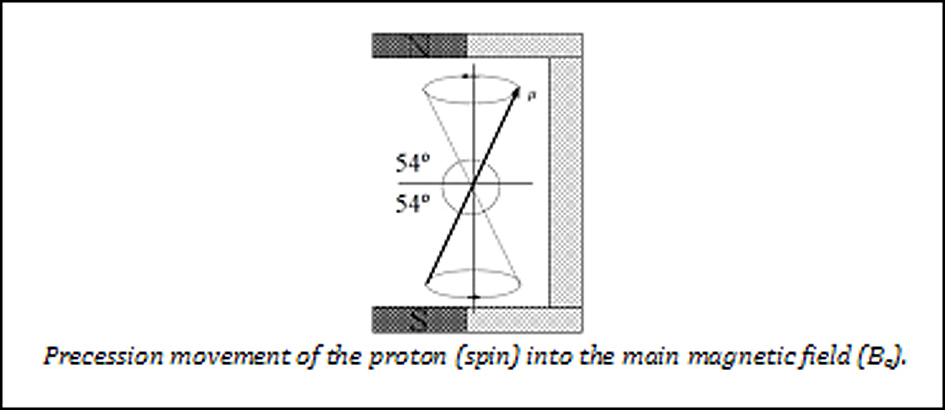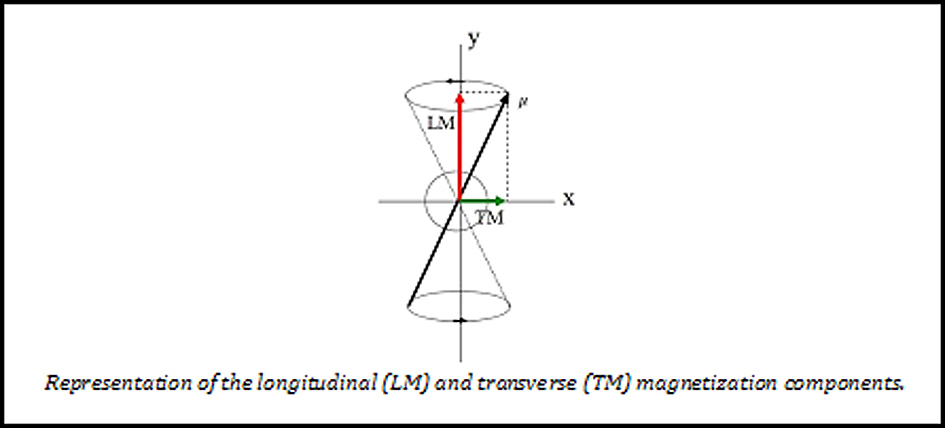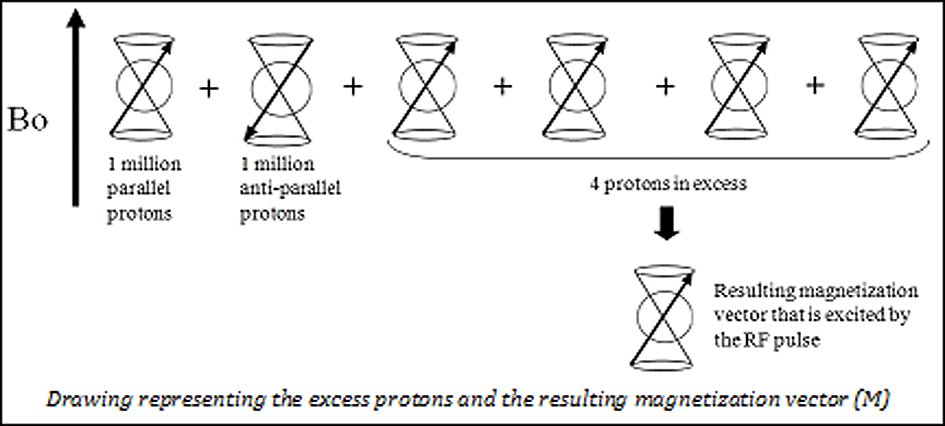## EFFECT OF A MAGNETIC FIELD ON THE PROTONS

As protons (spins) are spinning electrically charged particles, they behave as small magnets generating a magnetic field called a magnetic moment. This is represented by a magnetization vector "μ".

When the protons are placed into a magnetic field (Bo), they abandon their random orientation to align with it. But protons will resist the torque exerted by the magnet and rotate around the Bo field like a toy top that wobbles as it spins. So the magnetic vector (μ) describes a double cone at a rate frequency called precession frequency or resonance frequency (wo). It is proportional to the main magnetic field (Bo) and to the gyro-magnetic constant for each nucleus (g). Thus: wo= gBo

For biological tissues subjected to a field of 0.5 T the wo is 21.25 MHz, for 1T it is 42.5 MHz, and for 1,5T it is 63. 75 MHz.

The magnetization vector (μ) can be decomposed into two components: a longitudinal component (LM) and a transverse component (TM).

According to quantum mechanics, protons can have only two energy states at any given point: a low level of energy (parallel state) and a high level of energy (anti-parallel state). The magnetization vector of the parallel protons points in the same direction as the main magnetic field (Bo), and the magnetization vector of the anti-parallel protons points in the opposite direction. The separation between the two energy levels, parallel and anti-parallel, is proportional to Bo, being three times higher in a 1.5 T field than in a 0.5 T field. Consequently, the signal is better in a higher magnetic field.

The spins or protons are continuously oscillating between these two states of energy and tend to equilibrium. But there is always an excess of parallel protons (for a field of 0.5 T, there are 2 protons per million). If there were two million protons, they would be distributed as follows: 1 million parallel, 1 million anti-parallel and an excess of 4 parallel protons. This excess of parallel protons causes a macroscopic magnetization vector called M. When an electromagnetic wave (RF pulse) with an energy similar to the difference between these two states of energy is administered, it is possible to force a transition from the low state of energy to the high state and to modify the direction of the magnetization vector M. When half of the parallel protons have moved from a low energy level (parallel) to a high energy level (anti-parallel), the longitudinal component of the magnetization vector (ML) disappears. Only in the case of all the protons passing to a high energy level, will the longitudinal magnetization component   reverse to negative (-ML).